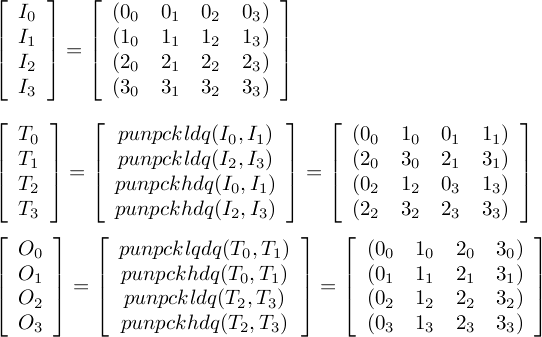# 4x4 integer matrix transpose in SSE2

The Intel SSE2 intrinsics has a macro _MM_TRANSPOSE4_PS which performs a matrix transposition on a 4x4 array represented by elements in 4 SSE registers. However, it doesn't work with integer registers because Intel intrinsics make a distinction between integer and floating point SSE registers. Theoretically one could cast and use the floating point operations, but it seems quite plausible that this will not round trip properly; for instance if one of your integer values happens to have the same value as a 32-bit IEEE denormal.

However it is easy to do with the punpckldq, punpckhdq, punpcklqdq, and punpckhqdq instructions; code and diagrams ahoy.

If we name the 4 input registers I0, I1, I2, and I3, then label their cooresponding elements as 0{0,1,2,3} and so on, then the transpose operation looks like this:When we are done, O{0,1,2,3} contains the all of the first, second, third, or fourth elements (resp) of the input vectors.

In Intel's intrinsics (also usable in at least GNU C++ and Visual C++), this can be expressed as:

```__m128i T0 = _mm_unpacklo_epi32(I0, I1);
__m128i T1 = _mm_unpacklo_epi32(I2, I3);
__m128i T2 = _mm_unpackhi_epi32(I0, I1);
__m128i T3 = _mm_unpackhi_epi32(I2, I3);

/* Assigning transposed values back into I[0-3] */
I0 = _mm_unpacklo_epi64(T0, T1);
I1 = _mm_unpackhi_epi64(T0, T1);
I2 = _mm_unpacklo_epi64(T2, T3);
I3 = _mm_unpackhi_epi64(T2, T3);
```

The diagram was done with latex2png, a handly little tool for generating images with LaTeX inputs.